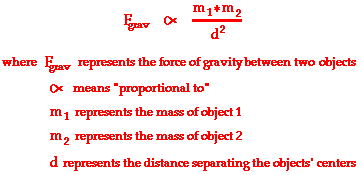Request a call back

how can we calculate the value of gravitational force in different places ??? Explain with some examples .
Asked by Pankaj Pal | 19 Jan, 2012, 10:15: AMExpert Answer
The force of gravitational attraction is directly dependent upon the masses of both objects and inversely proportional to the square of the distance that separates their centers. Newton's conclusion about the magnitude of gravitational forces is summarized symbolically asSince the gravitational force is directly proportional to the mass of both interacting objects, more massive objects will attract each other with a greater gravitational force. So as the mass of either object increases, the force of gravitational attraction between them also increases. If the mass of one of the objects is doubled, then the force of gravity between them is doubled. If the mass of both of the objects is doubled, then the force of gravity between them is quadrupled; and so on.

Since gravitational force is inversely proportional to the separation distance between the two interacting objects, more separation distance will result in weaker gravitational forces. So as two objects are separated from each other, the force of gravitational attraction between them also decreases. If the separation distance between two objects is doubled (increased by a factor of 2), then the force of gravitational attraction is decreased by a factor of 4 (2 raised to the second power). If the separation distance between any two objects is tripled (increased by a factor of 3), then the force of gravitational attraction is decreased by a factor of 9 (3 raised to the second power).

Answered by | 19 Jan, 2012, 10:24: AM

## Concept Videos

CBSE 11-science - Physics
Please answer this question.. The height of medium Earth orbit above the surface of the earth isAsked by varma.renu9481 | 17 Mar, 2023, 06:26: PMANSWERED BY EXPERT
CBSE 11-science - Physics
Asked by rajkumaeghogre975 | 10 Jan, 2023, 07:36: PMANSWERED BY EXPERT
CBSE 11-science - Physics
Asked by shivanipanchal6883 | 05 Jan, 2023, 02:44: PMANSWERED BY EXPERT
CBSE 11-science - Physics
Asked by ubarhandepramod | 21 Nov, 2022, 03:49: PMANSWERED BY EXPERT
CBSE 11-science - PhysicsAsked by ankurgoyal616 | 27 Sep, 2022, 03:07: PMANSWERED BY EXPERT
CBSE 11-science - Physics
Asked by halderishan008 | 30 Jul, 2022, 07:28: PMANSWERED BY EXPERT
CBSE 11-science - Physics
Asked by dibjotsingh770 | 04 Jul, 2022, 10:40: PMANSWERED BY EXPERT
CBSE 11-science - Physics
Asked by shmaahtabrez18 | 02 Sep, 2021, 12:23: PMANSWERED BY EXPERT
CBSE 11-science - Physics
Asked by Mp881337 | 06 Aug, 2021, 04:41: PMANSWERED BY EXPERT
CBSE 11-science - Physics
Asked by akshat.anamika | 01 Jun, 2021, 09:44: AMANSWERED BY EXPERT